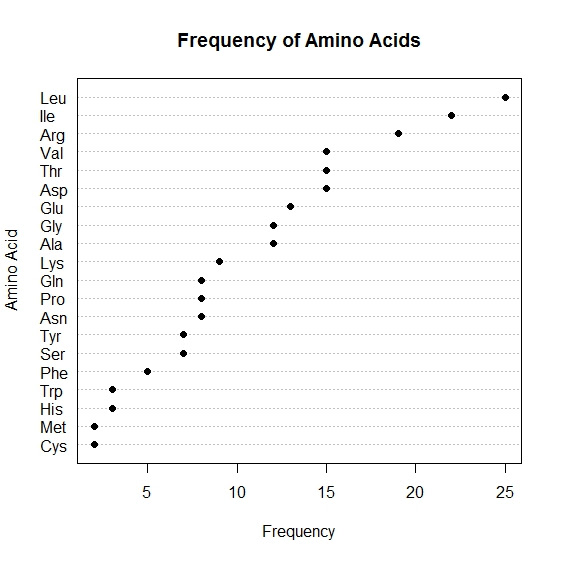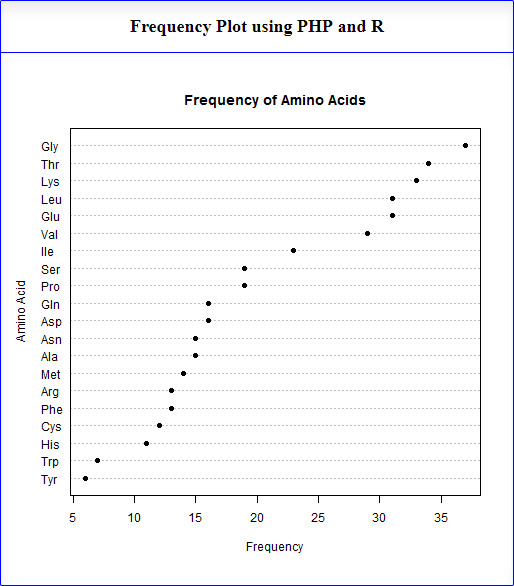# Frequency Plot of Protein Sequence using PHP and RA frequency plot is a graphical data analysis technique for summarizing the distributional information of a variable. The response variable is divided into equal sized intervals (or bins). The number of occurrences of the response variable is calculated for each bin. In this tutorial, the number of occurrences of each amino acids in the protein sequence (response variable) is calculated and sorted in ascending order.

The frequency plot then consists of:

Vertical Axis = Amino acids
Horizontal Axis = Frequencies of the amino acids

There are 4 types of frequency plots:

1. Frequency plot (absolute counts);
2. Relative frequency plot (convert counts to proportions);
3. Cumulative frequency plot;
4. Cumulative relative frequency plot.

The frequency plot and the histogram have the same information except the frequency plot has lines connecting the frequency values, whereas the histogram has bars at the frequency values.

### Frequency plot using PHP and R

In this tutorial, the programming language R, PHP, and BioConductor packages SeqinR & Biostrings are used to generate a frequency plot from the protein sequence. SeqinR is used to read or manipulate sequences, and Biostrings is used to convert sequence to array. The PHP language is used to execute Rscript at background using exec() function of PHP and the image generated through R (Rscript) is retrieved and displayed through the IMG HTML tag. The execution process acts similar to PHP/CGI or Perl/CGI. For generating a frequency plot, we need a protein sequence in .fasta|.fas file format as input. A simple protocol for generating a frequency plot is given below:

Step 3: Create an R script as given bellow using an ASCII editor (Eg. Notepad) and save it with .R file extension. Here args function is used to get path of the FASTA file formatted protein sequence through command line.

#### R Source Code (Freq.R)

args <- commandArgs(TRUE) fas_file <- args library("seqinr") library("Biostrings") seqfile <- read.fasta(file = fas_file) fastaseq <- seqfile[] seqstring <- c2s(fastaseq) seqstring <- toupper(seqstring) seqchar <- s2c(seqstring) tab <- table(seqchar) taborder <- tab[order(tab)] names(taborder) <- aaa(names(taborder)) png(filename="freq.png", width=500, height=500) dotchart(taborder, pch=19, main="Frequency of Amino Acids", xlab="Frequency", ylab="Amino Acid") dev.off()

#### Program Output